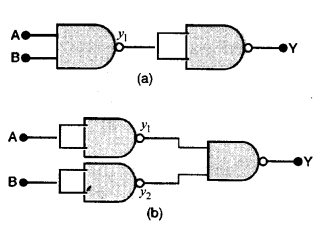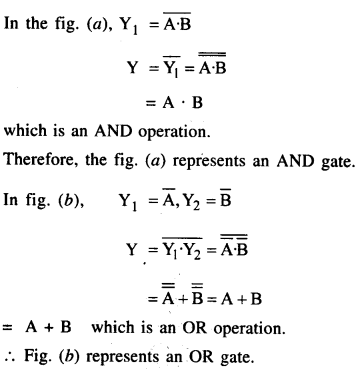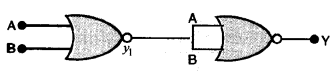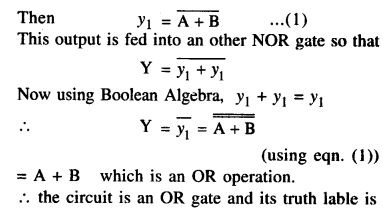# NCERT Solutions Class 12 Physics Chapter-14 (Electronics Devices)

NCERT Solutions Class 12 Physics from class 12th Students will get the answers of Chapter-14 (Electronics Devices) This chapter will help you to learn the basics and you should expect at least one question in your exam from this chapter.
We have given the answers of all the questions of NCERT Board Physics Textbook in very easy language, which will be very easy for the students to understand and remember so that you can pass with good marks in your examination.

Class 12 Physics

Chapter-14 (Electronics Devices)

Questions and answers given in practice

Chapter-14 (Electronics Devices)

Question 1.

In an n-type silicon, which of the following statement is true :
(a) Electrons are majority carriers and trivalent atoms are the dopants.
(b) Electrons are minority carriers and pentavalent atoms are the dopants.
(c) Holes are minority carriers and pentavalent atoms are the doplants.
(d) Holes are majority carriers and trivalent atoms are the dopants.

(c) ‘Holes are minority carriers and pentavalent atoms are the dopants in n type semiconductor.’

Question 2.
Which of the statements given in Exercise 1 is true for p-type semiconductors ?

(d) Holes are majority carriers and trivalent atoms are the dopants in p-type semiconductors.

Question 3.
Carbon, silicon and germanium have four valence electrons each. These are characterised by valence and conduction bands separated by energy band gap respectively equal to (Eg)c, (Eg)si and (Eg)Ge-
Which of the following statements is true ?
(a) (Eg)Si < (Eg)Ge < (Eg)c
(b)(E)c<(Eg)Ge>(Eg)si
(c) (Eg)c > (Eg)si > (Eg)Ge
(d) (Eg)c = (Eg)si = (Eg)Ge

(C) (Eg)c > (Eg)Si > (Eg)Ge. Energy band gap is maximum in carbon and least in germanium among the given elements.

Question 4.
In an unbiased p-n junction, holes diffuse from the p-region to n-region because
(a) free electrons in the n-region attract them.
(b) they move across the junction by the potential difference.
(c) hole concentration in p-region is more as compared to n-region.
(d) All the above.

(c) hole concentration in p-region is more as compared to n-region because hole diffusion takes place from higher concentration to lower concentration.

Question 5.
When a forward bias is applied to a p-n junction, it
(a) raises the potential barrier
(b) reduces the majority carrier current to zero
(c) lowers the potential barrier
(d) none of the above.

(c) lowers the potential barrier by cancelling the depletion layer.

Question 6.
For transistor action, which of the following statements are correct :
(a) Base, emitter and collector regions should have similar size and doping concentrations.
(b) The base region must be very thin and lightly doped.
(c) The emitter junction is forward biased and collector junction is reverse biased.
(d) Both the emitter junction as well as the collector junction are forward biased.

(b) and (c) : The base region must be very thin, lightly doped and the emitter junction is forward biased whereas collector junction is reverse biased to avoid unnecessary diffusion of charge carrier in the base and also for proper amplification.

Question 7.
For a transistor amplifier, the voltage gain :
(a) remains constant for all frequencies.
(b) is high at high and low frequencies and constant in the middle frequency range.
(c) is low at high and low frequencies and constant at mid frequencies.
(d) None of the above.

(c) is low at high and low frequencies and constant at mid frequencies as per frequency response of a transistor.

Question 8.
In half-wave rectification, what is the output frequency if the input frequency is 50 Hz. What is the output frequency of a full-wave rectifier for the same input frequency.

A half wave rectifier rectifies only one half cycle of input A.C.
.’. frequency of the output A.C.
= frequency of input A.C. = 50 Hz A full wave rectifier rectifies both halve cycles
of the A.C. input
.’. frequency of output A.C. = 2 x frequency of input A.C. = 2 x 50 = 100 Hz

Question 9.
For a CE-transistor amplifier, the audio signal voltage across the collector resistance of 2 kQ is 2 V. Suppose the current amplification factor of the transistor is 100, find the input signal voltage and base current, if the base resistance is 1 kΩ.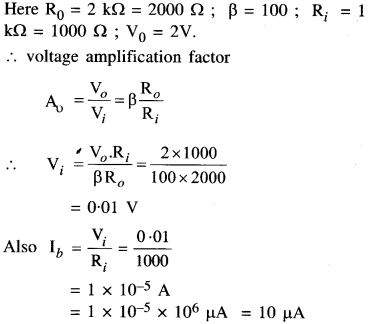Question 10.

Two amplifiers are connected one after the other in series (cascaded). The first amplifier has a voltage gain of 10 and the second has a voltage gain of 20. If the input signal is 0.01 volt, calculate the output ac signal.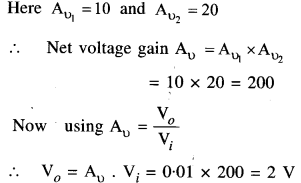Question 11.

A p-n photodiode is fabricated from a semiconductor with bandgap of 2.8 eV. Can it detect a wavelength of 6000 nm ?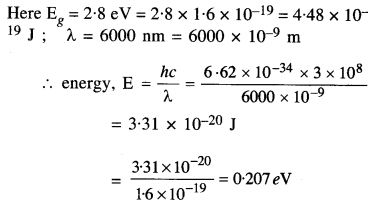Since the energy of the light photon is less than the bandgap energy of the p-n diode, it can not be detected.

Question 12.
The number of silicon atoms per m3 is 5 x 1028. This is doped simultaneously with 5 x 1022 atoms per mof Arsenic and 5 x 1020 per m3 atoms of Indium. Calculate the number of electrons and holes. Given that ni = 1.5 x 1016 m-3. Is the material n-type or p- type ?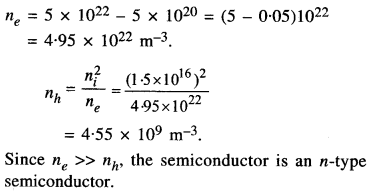Question 13.

In an intrinsic semiconductor, the energy gap Eg is 1.2 eV. Its hole mobility is much smaller than electron mobility and independent of temperature. What are the ratio between conductivity at 600 K and that at 300 K? Assume that the temperature dependence of intrinsic carrier concentration ni is given by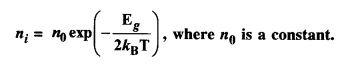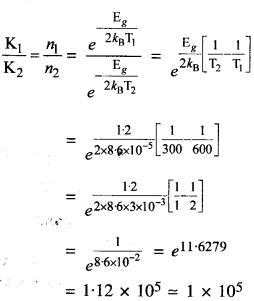Thus, conductivity of a semiconductor increases with rise in temperature.

Question 14.
In a P-n junction diode, the current I can be expressed as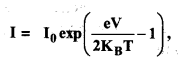where I0 is reverse saturation current. V is the voltage across the diode and is positive for forwarding bias and negative for reverse bias, and I is the current through the diode, Kis the Boltzmann constant (8.6 x 10-5 eV/K) and T is the absolute temperature. If for a given diode I0 = 5 x 10-12 A and T = 300 K, then
(a) What will be the forward current at a forwarding voltage of 0.6 V?
(b) What will be the increase in the current if the voltage across the diode is increased to 0.7 V?
(c) What is the dynamic resistance?
(d) What will be the current if reverse bias voltage changes from 1 V to 2 V?

The statement of the given question is incorrect. The relation should be

Question 15.
You are given the two circuits as shown in Figure. Show that circuit (a) acts as OR gate while circuit (b) acts as AND gate.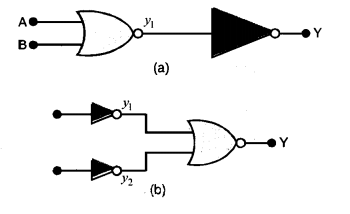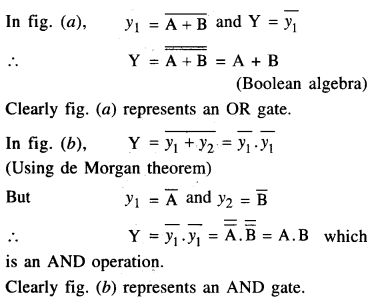Question 16.

Write the truth table for a NAND gate connected as given in Fig. Hence identify the exact logic operation carried out by these circuits.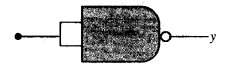The NAND gate shown in the truth table has only one input. Therefore, the truth table is

 A A y =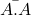0 0 1 1 1 0
 A Y 0 1 1 0

Question 17.
You are given two circuits as shown in Fig., which consist of NAND gates. Identify the logic operation carried out by the two circuits.